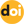The peer-reviewed scientific and technology journal. ISSN: 0204-3327

# The Effect of the Importance Function Resolution on the Accuracy of Calculating the Functionals of the Neutron Kinetics in Water Critical Assemblies by Monte Carlo Methodhttps://doi.org/10.26583/npe.2023.2.01

### UDC: 621.039.51

The paper considers a computational study of the importance function effect on the accuracy of calculating the effective fraction of delayed neutrons, βeff, and generation time of instantaneous neutrons using the MCU Monte Carlo code based on the example of three criticality experiments from the ICSBEP handbook.

In the MCU code, the importance function has a piecewise constant form: the computational model is broken down into a finite number of registration objects, and the neutron importance is calculated in each. The obtained importance values are used then to calculate the kinetic functionals due to which the calculation accuracy for the latter depends on the resolution.

Three types of the importance function spatial partition (axial, radial, combined) have been studied.

The numerical simulation results have shown that the axial component of the neutron importance function in all experiments has practically no effect on the calculation accuracy for βeff and Λ: the difference between the obtained values is less than 1 %.

The radial component has a notable effect (of up to 15.9 %) on the Λ calculation accuracy while having almost no effect on the βeff estimate. Using combined partition, as compared with radial partition, improves the calculation accuracy insignificantly (< 1%).

1. Sobol I.М. Numerical Monthe Carlo Methods. Мoscow. Nauka Publ., 1973, 312 p. (in Russian).
2. Voevodin V.V., Voevodin Vl.V. Parallel Computing. Saint-Petersburg. BHV-Petersburg Publ., 2002, 608 p. (in Russian).
3. Andrews G.R. Fundamentals of Multithreaded, Parallel and Distributed Programming. Moscow. Williams Publ., 2003, 512 p. (in Russian)
4. Lewins J.R. The Use of the Generation Time in Reactor Kinetics. Nuclear Science and Engineering. 1960, v. 7, pp. 122-126; DOI: https://doi.org/10.13182/NSE60-A29080 .
5. Kipin J.R. Physical Fundamentals of Nuclear Reactor Kinetics. Мoscow. Atomizdat Publ., 1967, 431 p. (in Russian).
6. Hetrick D.L. Dynamics of Nuclear Reactors. Мoscow. Atomizdat Publ., 1975, 400 p. (in Russian).
7. Kalugin M.A., Oleynik D.S., Shkarovsky D.A. Overview of the MCU Monte Carlo Software Package. Annals of Nuclear Energy. 2015, v. 82, pp. 54-62; DOI: https://doi.org/10.1016/j.anucene.2014.08.032 .
8. Gurevich M.I., Kalugin M.A., Oleynik D.S., Shkarovsky D.A. Estimation of Some Neutron Physics Characteristics by Monte Carlo Method Using the Importance Function. Annals of Nuclear Energy. 2019, v. 130, pp. 388-393; DOI: https://doi.org/10.1016/j.anucene.2019.02.047 .
9. Brun E., Damian F., Diop C.M., Dumonteil E., Hugot F.X., Jouanne C., Zoia A. TRIPOLI-4®, CEA, EDF and AREVA Reference Monte Carlo Code. Annals of Nuclear Energy. 2014, pp. 151-160; DOI: https://doi.org/10.1016/j.anucene.2014.07.053 .
10. Hendricks J.S. et al. MCNPX User’s Manual, Version 2.5.0. LA-UR-05-2675. – 2005.
11. Gurevich M.I., Oleynik D.S.,Shkarovsky D.A. Adaptation of the MCU-PD program to parallel computing on multiprocessor computers. VANT. Ser. Fizika Yadernykh Reaktorov. 2009, iss. 4, pp. 66-77 (in Russian).
12. Kalugin M.A., Oleynik D.S., Sukhino-Khomenko E.A. Evaluation of the Systematic Error of Monte Carlo Calculations of Neutron-Physical Properties Using a Multiprocessor Computer. Atomic Energy. 2011, v. 111 (2). pp. 79-85; DOI: https://doi.org/10.1007/s10512-011-9457-6 .
13. International Handbook of Evaluated Criticality Safety Benchmark Experiments, NEA/NSC/DOC(95)03, 2002.
14. Zolotukhin V.G., Majorov L.V. Evaluation of Reactor Criticality Parameters by the Monte Carlo Method. Moscow. Energoatomizdat Publ., 1984, 120 p. (in Russian).
15. Brown F.B., Kiedrowski B.C., Wilson P.P.H. Adjoint-Weighted Tallies for K-Eigenvalue Calculations with Continuous-Energy Monte Carlo. Nuclear Science and Engineering. 2011. v. 168 (3). pp. 226-241; DOI: https://doi.org/10.13182/NSE10-22 .
16. Oleynik D.S. Monte Carlo Calculation of Weakly Coupled Systems. Atomic Energy. 2005, v. 99. iss. 4. pp. 694-701; DOI: https://doi.org/10.1007/s10512-006-0002-y .
17. Brown F.B., Carney S.E., Kiedrowski B.C., Martin W.R. Fission Matrix Capability for MCNP, Part I – Theory. Mathematics & Computation. 2013, Sun Valley, ID, Report LA-UR-13-20429, Talk LA-UR-13-23152; DOI: https://doi.org/10.1051/snamc/201403502 .
18. Gurevich M.I., Shkarovsky D.A. Calculation of Neutron Transfer by the Monte Carlo Method According to the MCU Program. Study guide. Moscow. NRNU MEPhI Publ., 2012, 154 p. (in Russian).
19. Gurevich M.I., Shkarovsky D.A. Calculation of Neutron Transfer by the Monte Carlo Method According to the MCU Program in Examples. Study guide. Moscow. MIPT Publ., 2018, 160 p. (in Russian).
20. Mihajlov G.A., Rogazinskij S.V. Monte Carlo Weight Methods for Approximate Solution of the Nonlinear Boltzmann Equation. Sibirsky Matematichesky Zhurnal. 2002, v. 43, iss. 3, pp. 496-503; DOI: https://doi.org/10.1023/A:1015467719806 (in Russian).
21. Mihajlov G.A. Optimization of Monte Carlo Weight Methods. Moscow. Nauka Publ., 1987, 240 p. (in Russian).

Link for citing the article: Arkhangelsky D.M., Daichenkova Yu.S., Kalugin M.A., Oleynik D.S., Shkarovsky D.A. The Effect of the Importance Function Resolution on the Accuracy of Calculating the Functionals of the Neutron Kinetics in Water Critical Assemblies by Monte Carlo Method. Izvestiya vuzov. Yadernaya Energetika. 2023, no. 2, pp. 5-13; DOI: https://doi.org/10.26583/npe.2023.2.01 (in Russian).